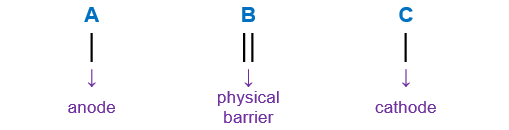# Problem: Write the half-reactions as they occur at each electrode and the net cell reaction for this electrochemical cell containing copper and silver: Cu(s) | Cu2+(aq) || Ag+(aq) | Ag(s)Anode?Cathode?Net cell reaction?

###### FREE Expert Solution

We’re being asked to anode, cathode, and net cell reaction for the given cell notation below:

Cu(s) | Cu2+(aq) || Ag+(aq) | Ag(s)

When writing a cell notation, we use the following format – “as easy as ABCRecall the mnemonics LEO GER.

87% (403 ratings)###### Problem Details

Write the half-reactions as they occur at each electrode and the net cell reaction for this electrochemical cell containing copper and silver: Cu(s) | Cu2+(aq) || Ag+(aq) | Ag(s)

Anode?

Cathode?

Net cell reaction?

Frequently Asked Questions

What scientific concept do you need to know in order to solve this problem?

Our tutors have indicated that to solve this problem you will need to apply the Cell Notation concept. If you need more Cell Notation practice, you can also practice Cell Notation practice problems.